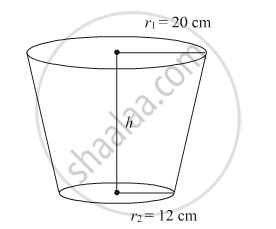# A bucket open at the top is in the form of a frustum of a cone with a capacity of 12308.8 cm3. The radii of the top and bottom circular ends are 20 cm and 12 cm, respectively. Find the height of the bucket and the area of metal sheet used in making the bucket. - Mathematics

A bucket open at the top is in the form of a frustum of a cone with a capacity of 12308.8 cm3. The radii of the top and bottom circular ends are 20 cm and 12 cm, respectively. Find the height of the bucket and the area of metal sheet used in making the bucket. (use π = 3.14)

#### Solution

Consider the following figure:Given:
Volume of the frustum is 12308.8 cm3.

Radii of the top and bottom are r= 20 cm and r= 12 cm, respectively.

Volume of the frustum is given by

V=1/3πh(r12+r22+r1r2)

12308.8×3=πh(202+122+20×12)

12308.8×3=πh(400+144+240)

12308.8×3=πh(784)

(12308xx3)/(3.14xx784)=h

(3920xx3)/784=h

15cm = h

Hence, height of the frustum is 15 cm.

Now,

Metal sheet required to make the frustum = Curved surface area + Area of the base of the frustum

Curved surface area of the frustum =π(r1+r2)l,  where l = sqrt(h^2+(r_1-r_2)^2)

l = sqrt(15^2+(20-12)^2)

=sqrt(225+64)

=sqrt289

=17 cm

Curved surface area of the frustum.

=π(20+12)17

=544×3.14

=1708.16 cm2

Area of the base =π122=144×3.14=452.16 cm2

∴ Metal sheet required to make the frustum=1708.16+452.16=2160.32 cm2

Concept: Heights and Distances
Is there an error in this question or solution?